## Selina Concise Mathematics Class 10 ICSE Solutions Chapter 24 Measures of Central Tendency (Mean, Median, Quartiles and Mode) Ex 24D

These Solutions are part of Selina Concise Mathematics Class 10 ICSE Solutions. Here we have given Chapter 24 Measures of Central Tendency (Mean, Median, Quartiles and Mode) Ex 24D.

Other Exercises

Question 1.
Find the mode of the following data:
(i) 7,9,8,7,7,6,8,10,7 and 6
(ii) 9,11,8,11,16,9,11,5,3,11,17 and 8
Solution:
(i) Mode = 7
because it occurs 4 times
(ii) Mode =11
because it occurs 4 times

Question 2.
The following table shows the frequency distribution of heights of 50 boys:Find the mode of heights.
Solution:
Mode is 122 because it occurs maximum times i.e its., frequency is 18.

Question 3.
Find the mode of following data, using a histogram: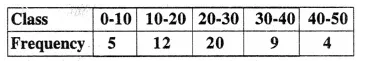Solution:
Mode class = 20 – 30
Mode = 24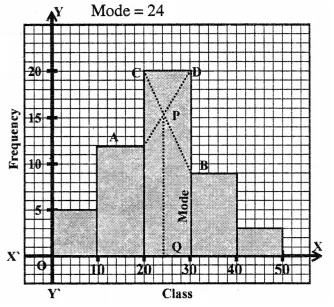We see in the histogram that line AD and CB intersect at P. Draw perpendicular Q to the horizontal x-axis. Which is the value of the mode = 24

Question 4.
The following table shows the expenditure of 60 boys on books. Find the mode of their expenditure:Solution:
Model class is = 30 – 35
and Mode = 34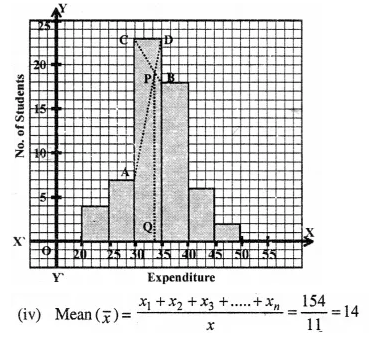We see in the histogram that line AD and CB intersect at P. Draw perpendicular Q to the horizontal axis. Which is the value of the mode.

Question 5.
Find the median and mode for the set of numbers 2,2,3,5,5,5,6,8 and 9.
Solution:
Median = $$\frac { 9 +1 }{ 2 }$$ = 5th term which is 5
Mode = 5, because it occurs in maximum times.

Question 6.
A boy scored following marks in various class tests during a term, each test being marked out of 20.
15,17,16,7,10,12,14,16,19,12,16.
(i) What are his modal marks ?
(ii) What are his median marks ?
(iii) What are his total marks ?
(iv) What are his mean marks ?
Solution:
Arranging the given data in ascending order : 7, 10,12, 12,14, 15,16,16, 16, 17,19.
(i) Mode = 16 as it occurs in maximum times.
(ii) Median= $$\frac { 11 +1 }{ 2 }$$ = 6th term which is 15
(iii) Total marks = 7 + 10+ 12+ 12+ 14+ 15+ 16 + 16+ 16+ 17+ 19= 154Question 7.
Find the mean, median and mode of the following marks obtained by 16 students in a class test marked out of 10 marks.
0,0,2,2,3,3,3,4,5,5,5,5,6, 6,7,8
Solution: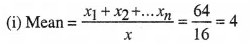(ii) Median = Mean of 8th and 9th term
= $$\frac { 4 +5 }{ 2 }$$ = $$\frac { 9 }{ 2 }$$ = 4.5
(iii) Mode = 5 as it occurs in maximum times.

Question 8.
At a shooting competition the score of a com-petitor were as given below :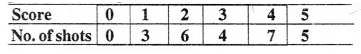(i) What was his modal score ?
(ii) What was his median score ?
(iii) What was his total score ?
(iv) What was his mean score ?
Solution: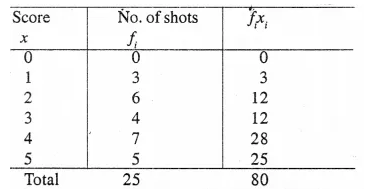(i) Modal score =4 as its frequency is 7, the maximum.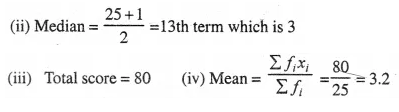Hope given Selina Concise Mathematics Class 10 ICSE Solutions Chapter 24 Measures of Central Tendency Ex 24D helpful to complete your math homework.

If you have any doubts, please comment below. Learn Insta try to provide online math tutoring for you.